﻿

### Transitive Partial Hyperbolic Flocks of Deficiency One

#### Abstract

artial hyperbolic flocks of deficiency one in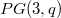$PG(3,q)$ are equivalent totranslation planes with spreads in$PG(3,q)$ admitting Baer groups of order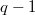$q-1$. In this article, we completely classify the associated derivabletranslation planes of order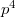$p^{4}$, for$p$ a prime, inducing a collineationgroup transitive on the partial flock. There are exactly two possiblenon-linear flocks and planes, those whose planes are the semifield of order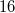$16$ with kernel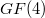$GF(4)$ and the plane of order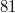$81$, due to Johnson and Pomareda.

DOI Code: 10.1285/i15900932v29n1p89

Keywords:
51E23; 51A40

Full Text: PDF